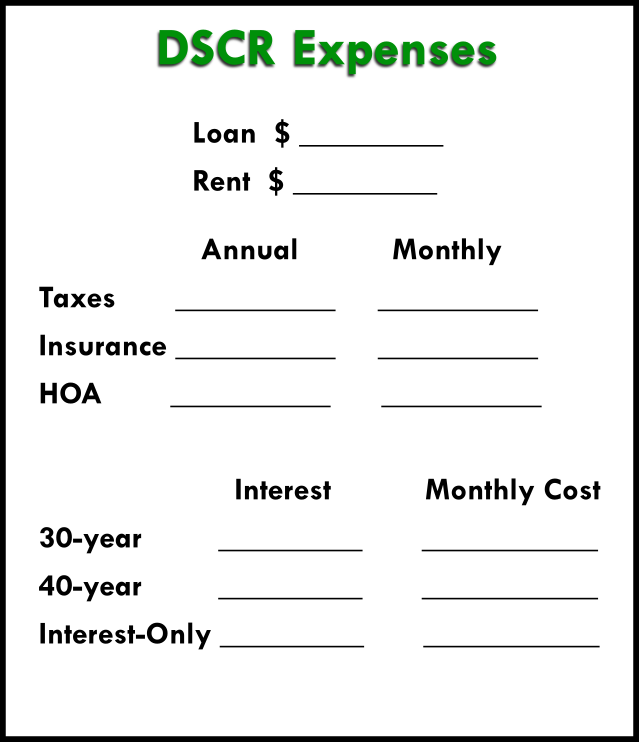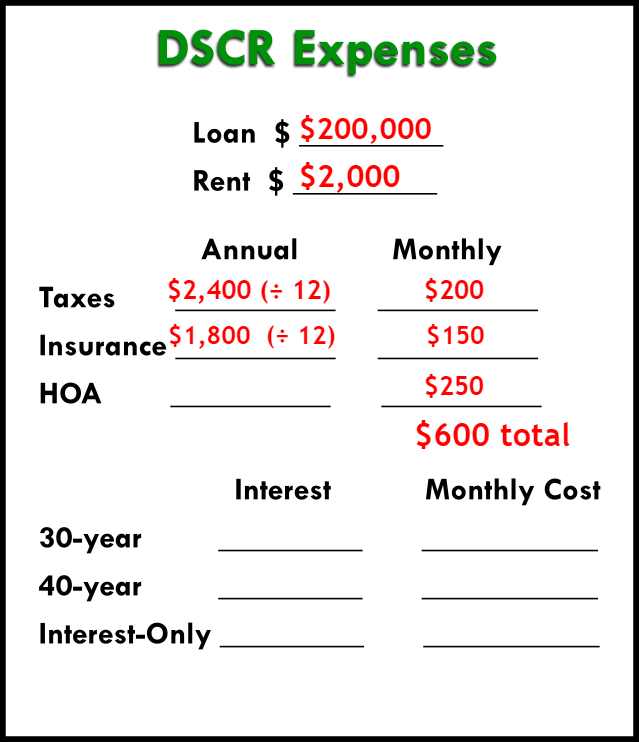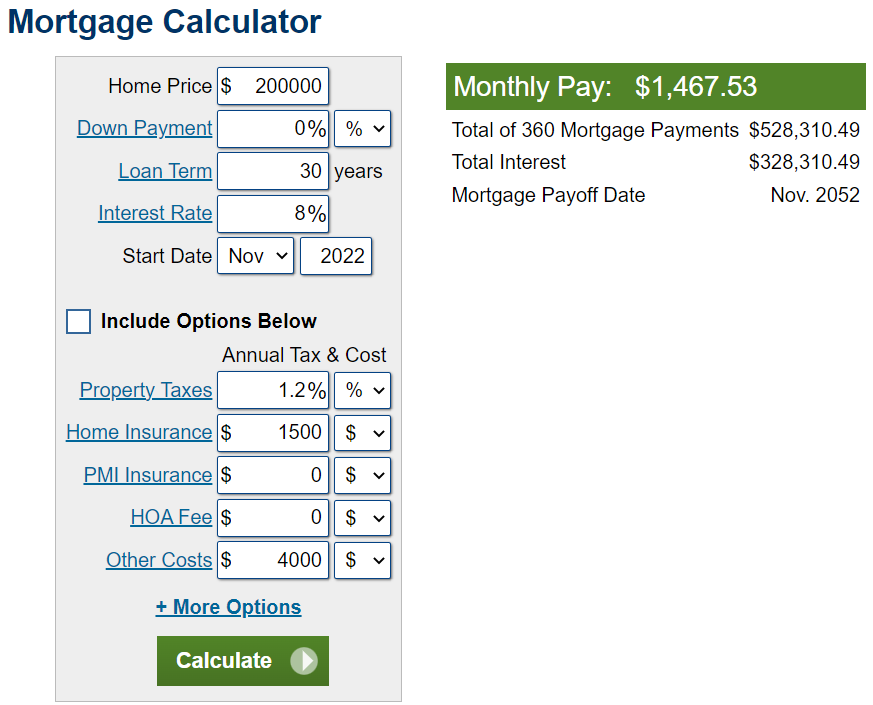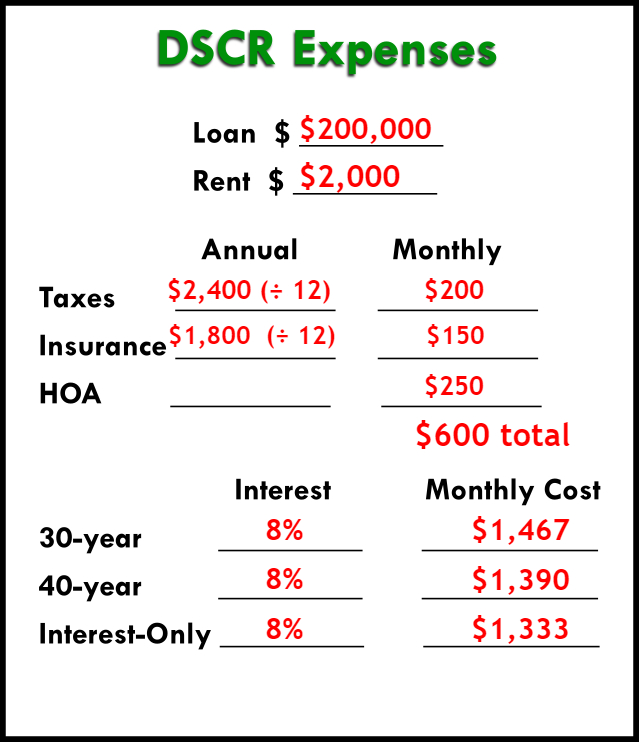## How to Calculate DSCR on Your Rental Property

Categories:

Here’s how to calculate a property’s DSCR (and what it means for your loan).

Don’t be intimidated by a DSCR loan. If the property cash flows, then you have a pretty good shot at getting approved.

And there’s a simple way to find out the cash flow of a rental property: the debt service coverage ratio.

This ratio is used by underwriters to determine if a property is positively cash flowing. It’s an important metric to understand if you want to maximize your leverage and get the most out of your investments.

Let’s go over how to calculate DSCR quickly and understand what it means for your property.

## What Is a DSCR in Real Estate?

First, let’s define what DSCR is. It’s a ratio that compares a property’s income to its expenses.

You calculate DSCR by dividing the property’s income (rents) by its expenses (monthly mortgage payment, taxes, insurance, and HOA if applicable). A ratio of greater than 1 means the property is cash flowing, which is what both you and your lender want to see.

The higher the ratio, the better the cash flow, and the more money in your pocket.

For a DSCR loan, the higher this ratio is, the better the terms your loan will have.

### How to Calculate Expenses & Income for a DSCR Loan

To find out the expenses your DSCR loan will consider, you’ll add together four items:

• Mortgage
• Property Tax
• Insurance
• HOA Fees

To find out the income, you’ll need to check out what rents are in the area for comparable properties.

## How to Calculate the DSCR

To give you a better understanding of how to calculate DSCR, let’s look at a quick example.

Let’s say we have a property with rents coming in at \$1,700 a month.

The monthly mortgage payment is \$1,290. Taxes are \$100/ month, insurance is \$100/month, and HOA is \$100 /month. Added together, this gives us \$1,590.

Now, to calculate the DSCR ratio, we divide the income (\$1,700) by the expenses (\$1,590). We get a ratio of 1.07.

This is great! The break-even point for a DSCR is a ratio of 1. Underwriters and lenders like to see a ratio of at least 1 to ensure that the property can take care of itself. Now lenders know you won’t need to take money out of your pocket to cover the expenses. This is assurance for them, making them more likely to approve the loan with good terms.

A 1.07 ratio means the property is positively cash flowing, and it’s a good investment.

### Example of a Low Ratio

But what if we could only charge \$1,500 in rent for this same property?

Let’s look at the impact of a decrease in rent. In this case, we’d calculate the DSCR ratio by dividing \$1,500 (income) by \$1,590 (expenses), which gives us 0.94. You’ll need an extra \$90 out-of-pocket just to breakeven.

This is less than 1, meaning the property is negatively cash flowing.

You need to estimate the rent on a property before you think about buying it. This property at \$1,500 wouldn’t be a good investment (and wouldn’t qualify for a good DSCR loan). But remember – the same property at \$1,700 rent would be a good investment.

Usually, the only time DSCR loans are used on a negatively cash-flowing property is when someone gets stuck with a property they can’t sell, and a little income on the property is better than none at all. It’s not wise to purchase a rental property that you know won’t cash flow from day 1.

## Negative DSCR Loans

You can still find a DSCR product for negative cash flow properties.

There are certain thresholds when you calculate DSCR loans. When you break these thresholds, you get a better rate. And better rates mean… more cash flow! Your monthly payments will lower.

Let’s go over what some of these thresholds will look like.

### Loans for a 1.25 DSCR

Say we have a property with \$1,590 worth of monthly expenses, which we can charge a \$2,000 rent on. Divide the rent by the expenses, and we get a DSCR of about 1.26.

One way of thinking of this is that the property is profiting 25% over the expenses. That’s good for the underwriter (and it’s good for you).

1.25 is a threshold for DSCR lenders. In the current market at the beginning of 2022, the rate for a 1.25 DSCR is around 7.25%.

### Rates for a Negative to 1 DSCR

If a property has negative cash flow, say 0.944, then the average interest rate would be 9+% on a DSCR loan.

For a breakeven ratio of 1, the typical interest rate right now would be more like 7.75%.

### The Difference

Anytime you can lower the rate, that’s cash flow that goes into your pocket.

The difference between a negative DSCR and a 1.25 is about \$220/month on your payment. Over the course of a year, that adds up to \$2,600. If you have 5 rental properties, that’s \$13,000/year. At 10 rental properties, it’s a \$26,000 difference!

If real estate investing is going to be your career or retirement plan, buying properties that you know will cash flow is vital. A couple hundred bucks a month can snowball into hundreds of thousands over time.

This is why it’s important to know how to calculate DSCR quickly when you’re looking at buying a new property. Never put a contract on a rental property when you’re not sure if the cash flow fits your goals.

## How to Calculate DSCR Quickly

To help keep the numbers straight when you calculate DSCR, you can download our free, simple DSCR calculator at this link.

If you have any other questions about how to calculate DSCR (or how to get a DSCR loan!), send us an email at Info@TheCashFlowCompany.com.

by

## Sample of a DSCR Loan Calculation: The Costs

Categories:

An important part of a DSCR loan is knowing your costs. Here’s an example DSCR loan calculation to help you out.

A DSCR loan will be a useful product in your real estate investing career. It requires no income verification and no work or investment history. These loans only require that the property’s income is the same (or higher than) the expenses.

Cash flow really is kind for DSCR loans. Here’s a breakdown of how to calculate the expenses to see if you qualify.

## Calculate a DSCR Loan Expenses

You can follow along with your DSCR loan calculator (free download here). We’ll fill out this form to show each step of a DSCR loan calculation.### Rent Income & Loan Amount

Firstly, you need to estimate your loan amount and your rent income. If you have a deal in front of you, you probably have a good idea of the loan amount you’ll need to be able to afford the property.

As for rent, you can get realistic amounts from online sources. Look at Zillow or Rent.com to find the market rate for rent in the property’s neighborhood.

Let’s keep it simple for our example and say our loan is \$200,000, and our rent income is \$2,000.

### What Expenses Count in a DSCR Loan?

We know our income (rent), but now we need to figure out our costs.

The expenses considered in a DSCR loan DO include:

Taxes

Insurance

HOA fees

Expenses NOT considered in a DSCR loan are things like:

Property management fees

Utilities

Maintenance

To estimate the taxes on the property, you could use a property tax calculator like this one. If you need an estimate on insurance, you can try this home insurance calculator. You can figure out HOA fees by contacting the HOA, if that applies to your property.

If any of these costs are charged annually, then you’ll need to divide by 12 to break it down into a monthly cost.

Let’s take a look at what information we have now for our example DSCR loan:### Calculating Loan Cost

Secondly, DSCR lenders will offer many types of the loans – fixed-rate mortgages, interest-only, ARMs, etc. You need to find what best fits you, and to do that, you’ll have to run all the numbers.

To calculate each of the amortized loans, you can use an amortization calculator like this one. Add in your information – loan amount, interest rate, and loan length.We’re going to use an 8% interest rate for our example, since that’s the anticipated average for next year.

In reality, each loan and lender will have a different interest rate. Additionally, the interest rate may fluctuate depending on your qualifications and DSCR. You can get this information from your lenders to plug into your calculator.

We’ll use three common loans for this example: a 30-year fixed, 40-year fixed, and interest only loan.

If we had a 30-year mortgage for \$200,000 at 8%, our monthly payment would be \$1,467.

For a 40-year fixed with the same info, payments would be \$1,390.

For interest-only, you can calculate the loan fairly simply yourself. Multiply the loan amount by the interest rate (e.g., 200,000 × .08 = 16,000). That gives you the yearly interest, then you divide it by 12 to get the monthly payment. For our example, that’s \$1,333.So what do you do with these numbers? How do you know which loan is best?

It depends on your priorities. To have the most cash flow, the lowest number is best (in this case, interest-only). If you need something that amortizes, a 30-year would probably be best.

But you don’t really know which loan will be best for you until you calculate the DSCR.

Watch the video here.

by